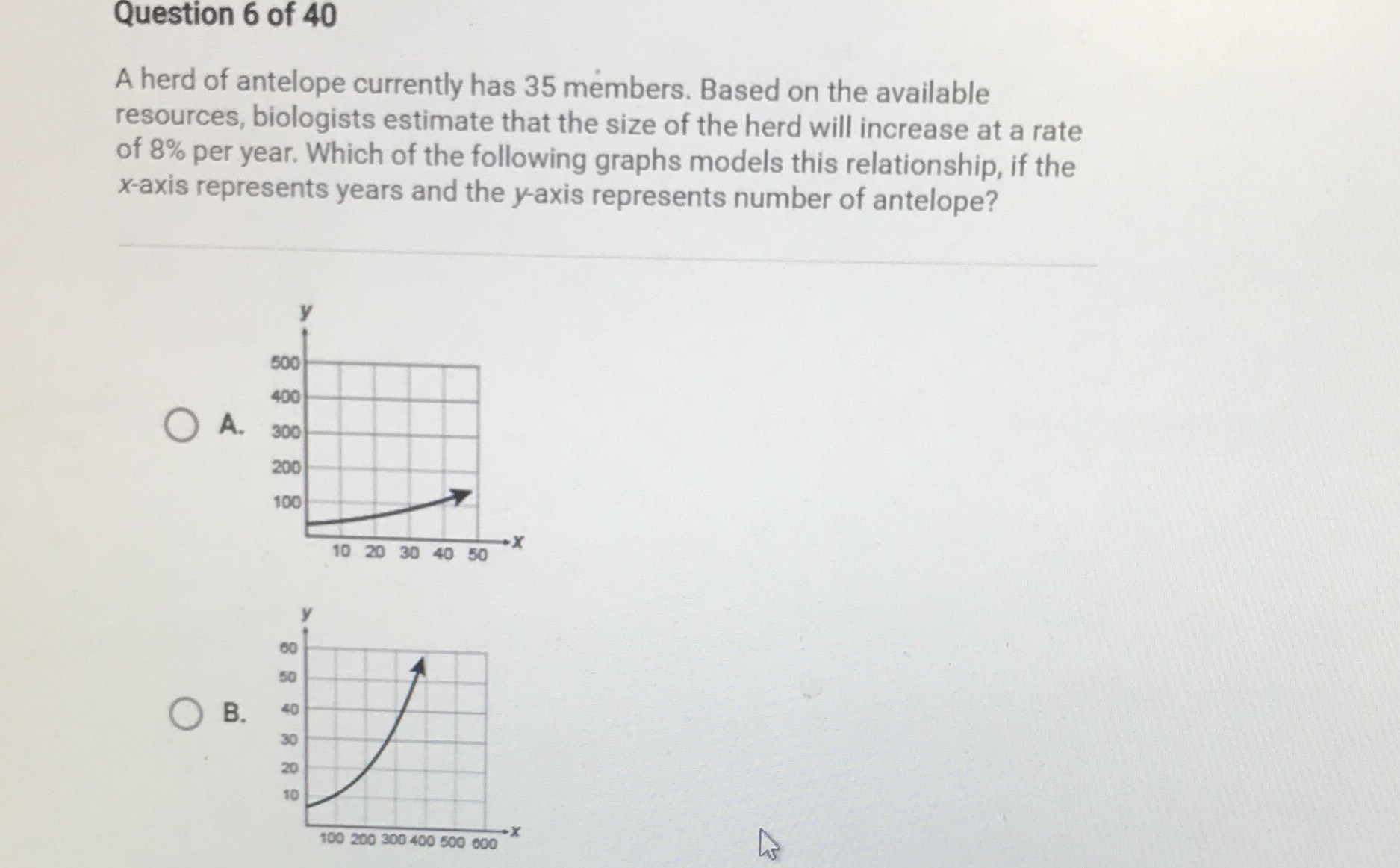### ¿Todavía tienes preguntas de matemáticas?

Pregunte a nuestros tutores expertos
Algebra
PreguntaA herd of antelope currently has $$35$$ members. Based on the available resources, biologists estimate that the size of the herd will increase at a rate of $$8 \%$$ per year. Which of the following graphs models this relationship, if the $$x$$ -axis represents years and the $$y$$ -axis represents number of antelope?